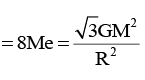Courses

# Test: Gravitation- 3

## 30 Questions MCQ Test Physics For JEE | Test: Gravitation- 3

Description
This mock test of Test: Gravitation- 3 for JEE helps you for every JEE entrance exam. This contains 30 Multiple Choice Questions for JEE Test: Gravitation- 3 (mcq) to study with solutions a complete question bank. The solved questions answers in this Test: Gravitation- 3 quiz give you a good mix of easy questions and tough questions. JEE students definitely take this Test: Gravitation- 3 exercise for a better result in the exam. You can find other Test: Gravitation- 3 extra questions, long questions & short questions for JEE on EduRev as well by searching above.
QUESTION: 1

### Four particles, each of mass M, move along a circle of radius R under the action of their mutual gravitational attraction. (Take that gravitational force between two point masses m1 and m2 separated by is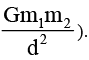The speed of each particle is

Solution: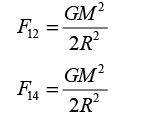The resultant of these two forces is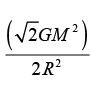.Now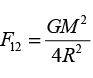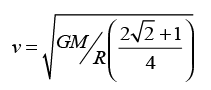QUESTION: 2

### At what altitude will the acceleration due to gravity be 25% of that at the earth’s surface (given radius of earth is R)?

Solution:

Force on the body placed on Earth's surface is
F=GMm/R2​
But, F=mg hence,
mg=GMm/R2
where, variables have their usual meanings.
gR2=GM
Now, force on the body at geo-potential  height say h (altitude) where the acceleration due to gravity is 25% of that at the earth's surface i.e.
25g/100​=g/4​
Hence, we can write
g/4​=GM/(R+h)2

g/4​= gR2​ /(R+h)2

(R+h)2=4R2
Taking roots for both sides we get
R+h=2R
h=R

QUESTION: 3

### A planet of mass M is revolving around sun in an elliptical orbit. If dA is the area swept in a time dt, angular momentum can be expressed as

Solution: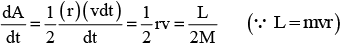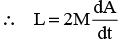QUESTION: 4

Let ω be the angular velocity of the earth’s rotation about its axis. Assume that the acceleration due to gravity  on the earth’s surface has the same value at the equator and the poles. An object weighed at the equator gives the same reading as a reading taken at a depth d below earth’s surface at a pole (d<<R) The value of d is

Solution:
QUESTION: 5

A straight rod of length L extends from x = a to x = L + a. Find the gravitational force exerted by it on a point mass m at x = 0 if the linear density of rod μ = A+ Bx2

Solution: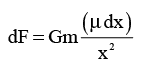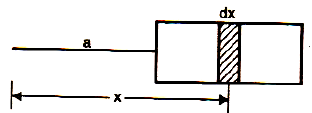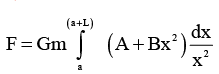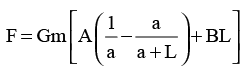QUESTION: 6

With what angular velocity the earth should spin in order that a body lying at 30° latitude may become weightless [R is radius of earth and g is acceleration due to gravity on the surface of earth]

Solution:

g' = acceleration due to gravity at latitude
g = acceleration due to gravity at poles
ω = angular velocity of earth
(θ) = latitude angle
Now,
g' = g - Rω^2 cos^2(θ)
As, g' = 0 (weightless):
g = Rω^2 cos^2(θ)
g = Rω^2 cos^2(30)
g = Rω^2 (3/4)
ω^2 = 4g/3R
ω = √(4g/3R)

QUESTION: 7

The work done in shifting a particle of mass m from centre of earth to the surface of earth is (0m at both places are fixed)

Solution: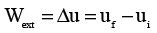Here u i = gravitational potential energy at the centre of earth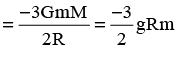u = gravitational potential energy at the surface of earth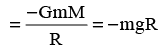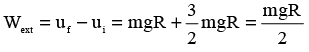QUESTION: 8

A man of mass m starts falling towards a planet of mass M and radius R. As he reaches near to the surface, he realizes that he will pass through a small hole in the planet. As he enters the hole, he sees that the planet is really made of two pieces a spherical shell of negligible thickness of mass 2M/3 and a point mass M/3 at the centre. Change in the force of gravity experienced by the man is

Solution: The gravitational force on the man when he was falling towards the planet was (GMm)/r^2. G here is the constant. M is mass of the whole planet ie M/3 + 2M/3. m is mass of the man. and r is the radius of the planet. once he has entered the hole in the planet. the force he was experiencing earlier due to the outer shell of the planet becomes 0. and now he experiences force only due to the point mass at the centre. this force is equal to (GMm)/3r^2. thus the change in force is initial - final force experienced which is equal to (2GMm)/3r^2. thus the answer.
QUESTION: 9

A particle is projected upward from the surface of earth (radius = R) with a speed equal to the orbital speed of a satellite near the earth’s surface. The height to which it would rise is

Solution: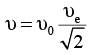(orbital speed υ0 of a satellite)

Near the earth’s surface is equal to 1/√2
times the escape velocity of a particle on earth’s surface) Now from conservation of mechanical energy: Decrease in kinetic energy = increase in potential energy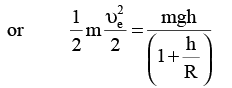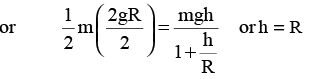QUESTION: 10

Two masses m1 & m2 are initially at rest and are separated by a very large distance. If the masses approach  each  other subsequently, due to gravitational attraction between them, their relative velocity of approach at a separation distance of d is :

Solution:

We use the energy balance
Initial potential energy equals final kinetic energy
Gm1​m2/d​​=1mv12​/2+1​mv22​/2
also, from the conservation of momentum we have
m1​v1​=m2​v2
or
v1​= ​m1​v1/ m2
Substituting this we get
v1​=√2Gm22​​​/d(m1​+m2​)
Similarly, we have
v2​= √2Gm12​​​/ d(m1​+m2​)
Now as velocities are in opposite direction their relative velocity is v1​−(−v2​)=v1​+v2
or
[2G(m1​+m2​)​​/ d]1/2

QUESTION: 11

The ratio of the energy required to raise a satellite to a height h above the earth to that of the kinetic energy of satellite in the orbit there is (R = radius of earth)

Solution:

Energy required to raise a satellite upto a height h: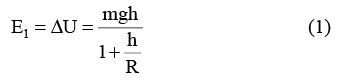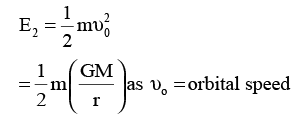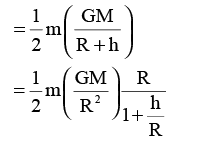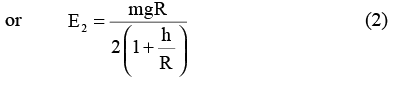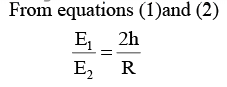QUESTION: 12

Two concentric shells of uniform density of mass M1 and M2 are situated as shown in the figure. The forces experienced by a particle of mass m when placed at positions A, B and C respectively are (given OA = p, OB = q and OC = r)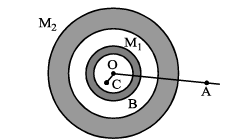Solution:

We know that attraction at an external point due to spherical shell of mass M is GM/r2 while at an internal point is zero. So, for particle at point C
OA = G (M1 + M2)m/p2
OB = G M1m/q2
OC = 0

QUESTION: 13

A spherical hole is made in a solid sphere of radius R. The mass of the original sphere was M.The gravitational field at the centre of the hole due to the remaining mass is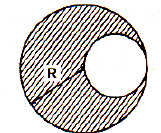Solution:

By the principle of superposition of fields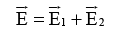Here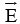= net field at the centre of hole due to entire mass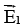= field due to remaining mass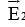= field due to mass in hole = 0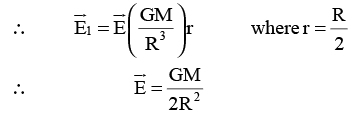QUESTION: 14

At what height above the earth’s surface does the accelerati on due to gravity fall to 1% of its value at the earth’s surface?

Solution: 1/(R+h)^2 =1/100(R)^2
Solve and you will get h=9R
QUESTION: 15

A ring has a total mass m but not uniformly distributed over its circumference. The radius of the ring is R. A point mass m is placed at the centre of the ring. Work done in taking away the point mass from centre to infinity is

Solution:

W = increase in potential energy of system

=Uf - Ui

=m (Vf - Vi)

(V = gravitational potential)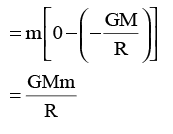Note: Even if mass is nonuniformly distributed potential at centre would be -GM/R

QUESTION: 16

If the radius of the earth be increased by a factor of 5, by what factor its density  be changed to keep the value of g the same?

Solution:

Since density = M/V and M = gr^2/G and V = 4/3πr^3
Therefore density = (3/4) * (g/πrG) and new density = (3/4)*(g/πr'G)
As r’ = 5r then the new density = ¾ * (g/5rGπ)
d’ = 1/5*3/4(g/πrG)
d'=1/5 d

QUESTION: 17

Imagine a light planet revolving around a very massive star in a circular orbit of radius R with a period of revolution T. If the gravitational force of attraction between the planet and the star is R−5/2 , then T2 is proportional to

Solution: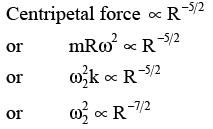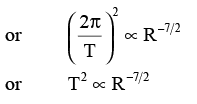QUESTION: 18

Two point masses of mass 4m and m respectively separated by d distance are revolving under mutual force of attraction. Ratio of their kinetic energies will be :

Solution:

Let’s say the velocity of ‘m’ is ‘v’ and velocoty of ‘4m’ is ‘u’
Therefore, mv = 4mu ---- (Given)
So, v = 4u
Now, we know that kinetic energy = (1/2) mv2
Thus for the ratio, we need to calculate
(1/2 * m * (4u)2) / (1/2 * 4m * u2)
It turns out to be 4/1.
Which means the ratio of kinetic energies of both masses is 1:4

QUESTION: 19

If G is the universal gravitational constant and ρ is the uniform density of spherical planet.
Then shortest possible period of the planet can be

Solution:

The fastest possible rate of rotation of a planet is that for which the gravitational force on material at the equator just barely provides the centripetal force needed for the rotation. Let M be the mass of the planet R its radius and m the mass of a particle on its surface. Then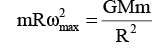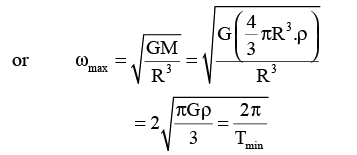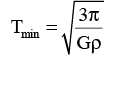QUESTION: 20

Four similar particles of mass m are orbiting in a circle of radius r is the same direction and same speed because of their mutual gravitational attractive force as shown in the figure. Speed of a particle is given by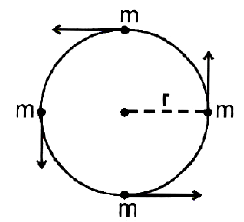Solution:

Net force is towards centre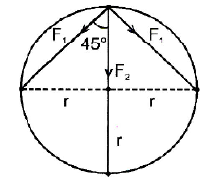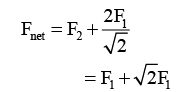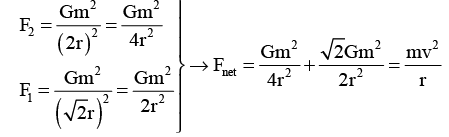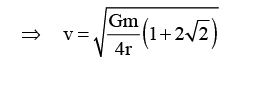QUESTION: 21

A planet is moving in an elliptical path around the sun as shown in figure. Speed of planet in positional P and Q are υ1 and υ2 respectively with SP = r1 and SQ = r2 , then υ12 is equal to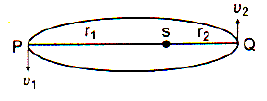Solution:

Angular momentum of planet about the sun is constant.

i.e. mυr sin θ = constant

At position P and Q, θ = 900 and m = mass of planet = constant

∴ υr = constant

QUESTION: 22

Consider two configurations of a system of three particles of masses m, 2m and 3m. The work done by external agent in changing the configuration of the system from figure (i) to figure (ii) is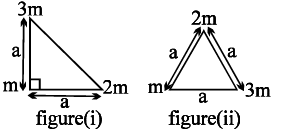Solution:
QUESTION: 23

Three solid spheres each of mass m and radius R are released from the position shown in figure. The speed of any sphere at the time of collision would be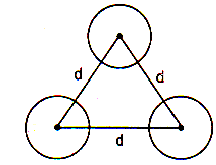Solution:

From conservation of mechanical energy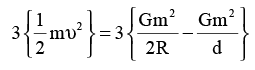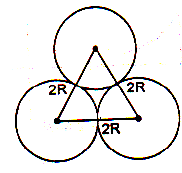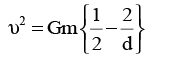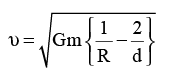QUESTION: 24

Three particles P, Q and R placed as per given figure. Masses of P, Q and R are √3 m, √3 m and m respectively. The gravitational force on a fourth particle ‘S’ of mass m is equal to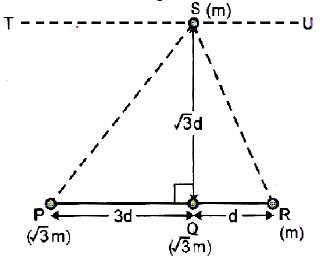Solution:

in horizontal direction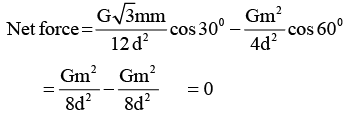in vertical direction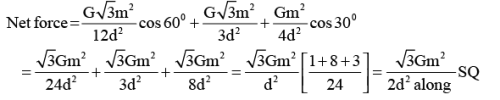QUESTION: 25

How much deep inside the earth should a man go so that his weight becomes one fourth of that at a point which is at a height R above the surface of earth .

Solution: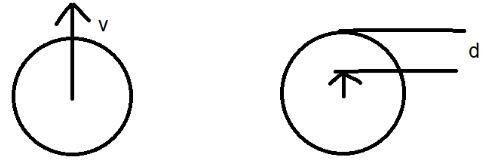g=GM/r2                                                g1=GMr/R3
we are given that,
GMr/R3 =(1/4) GM/(R+R)2
=>r/R3=(1/4).(1/4R2)
=>r=R/6
Therefore, d=R-r=15R/16

QUESTION: 26

Three identical stars of mass M are located at the vertices of an equilateral triangle with slide L. The speed at which they will move if they all revolve under the influence of one another’s gravitational force in a circular orbit circumscribing the triangle while still preserving the equilateral triangle

Solution:
QUESTION: 27

The period of revolution of planet A around the sun is 8 times that of B. The distance of A from the sun is x times that of B from the sun. Find x.

Solution:

Since we know that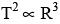and ATQ TA = 8TB so,
⇒ (Ta/Tb)2 = (Ra/Rb)3
⇒ 64 = x3
⇒ x = 4

QUESTION: 28

n – particles each of mass m0 are placed on different corners of a regular polygon of edge length a. The distance between vertex and centre of polygon is r0 . The gravitational potential at the centre of the polygon is

Solution: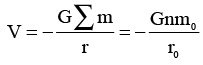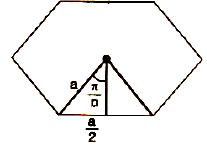QUESTION: 29

If an artificial satellite is moving in a circular orbit around the earth with a speed equal to half the magnitude of the escape velocity form the earth, the height of the satellite above the surface of the earth is

Solution: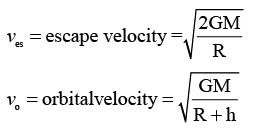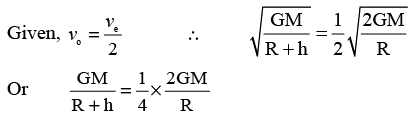on solving we get;h = r

QUESTION: 30

A uniform ring of mass M and radius R is placed directly above uniform sphere of mass 8M and of same radius R. The centre of the ring is at a distance of d = √3R from the centre of sphere. The gravitational attraction between the sphere and the ring is

Solution:

Gravitational field due to the ring  at a distance d = √3R on its axis is: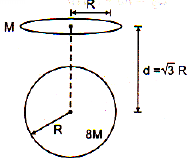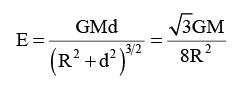force on sphere